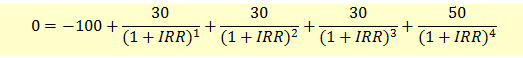# Internal Rate of Return

IRR is the case of a discount rate that equalizes the present value of cash inflows with present value of cash outflows. Within the context of a net present value analysis, when the cash inflows and outflows are known, IRR will be the rate that causes the NPV to equal zero.

The formula for IRR is as follows:In our example, the IRR will be calculated as follows:Solving the above equation, we get IRR = 13.663%.

This is solved by trial and error method. In Excel, you can use Goal Seek to solve for IRR.

 Calculator Usage We can solve the IRR problems using the official BA II Plus calculator as follows:Step 1: Enter Cash FlowsBefore you enter the new cash flows, clear the previous work by pressing the keys CF, 2ND, CLR WORK. Now you are ready to enter the cash flows. You should see CF0 on screen. Enter the cash flows as follows:Initial investment         100[+/-][ENTER]         CF0 = -100Period 1 Cash Flow    [↓]                          CF1 = 30Period 2 Cash Flow    [↓][↓]                      CF2 = 30Period 3 Cash Flow    [↓][↓]                   CF3 = 30Period 4 Cash Flow    [↓][↓]                   CF4 = 50Step 2: Compute IRRCompute IRR              [IRR][CPT]                             NPV = 13.663